# Isaac Newton's First Law of Motion

The First Law is concerned with changes in velocity caused by non-zero net forces.

Isaac Newton stated three laws of motion; the first law deals with forces and changes in velocity. For just a moment, let us imagine that you can apply only one force to an object. That is, you could choose push the object to the right or you could choose to push it to the left, but not to the left and right at the same time, and also not up and to the right at the same time, and so on.

Under these conditions the first law says that if an object is not pushed or pulled upon, its velocity will naturally remain constant. This means that if an object is moving along, untouched by a force of any kind, it will continue to move along in a perfectly straight line at a constant speed.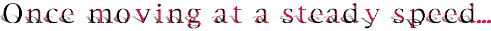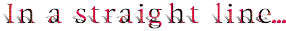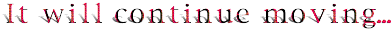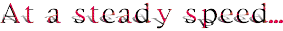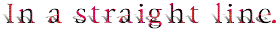In the animation above we see an object (blue box) that is already moving along when we encounter it. As long as we do not push or pull it, as long as it does not run into something else or rub against something else, it will continue to move in a straight line at constant speed. It will continue to do this on its own without the need for any influence from other agents.

The first law also means that if an object is standing still and is not contacted by any forces, it will continue to remain motionless. Actually, a motionless object is just a special case of an object that is maintaining constant velocity. Its velocity is constantly 0 m/s.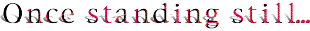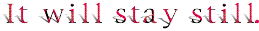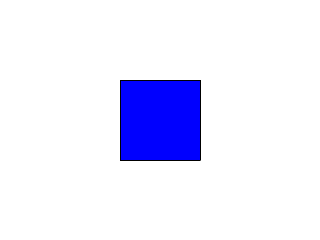In the scene above we see an object (blue box) that is standing still. On its own it will continue to stand still as long as we do not influence it with a force, that is, with an unbalanced, non-zero force.

 Understand that we are saying if no outside force is applied, the velocity of the object (which is its speed and direction) will remain constant. An object traveling in a straight line at constant speed has a constant velocity. An object standing still has a constant velocity too. Its constant velocity is zero in value. In order to change either of these examples of constant velocity, you need to apply a non-zero force to the object. Then, when the force is applied, the velocity will change, and the object experiences an acceleration.

Several forces can act at once on the object.

Now, what about if there is more than one force on the object? You really can push an object, say, to the left and down at the same time, so, what happens then?

Under these conditions we must realize that a group of forces on an object adds up so that all the forces appear to the object as one force. This one force that is the sum of all the forces is called the net force. (You might want to read some information on net force. It is here.) The word net in this context means total. It is this net force that may change the velocity of the object. Let us look at some examples.

Imagine that two forces act at the same time on an object. One is a very strong force to the left, and the other is a weaker force to the right. These two forces add up to one net force. Since the force to the left is stronger, the net force is to the left.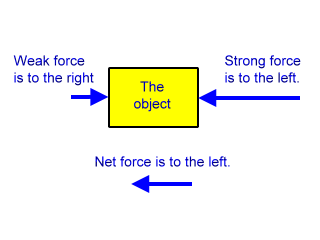This net force to the left will cause the velocity of the object to change. The object experiences this one net force as if this was the only force pushing it, although, actually, there are two separate forces present. Next let us see what happens when two forces act, but they are equal in strength.

Imagine that two forces, one to the right and one to the left, push on an object, and imagine that the two forces are the same size. These two forces add up as before, but this time one of them does not overpower the other. They cancel each other out. So, in this example the net force is zero. It is as though no forces were really acting on the object.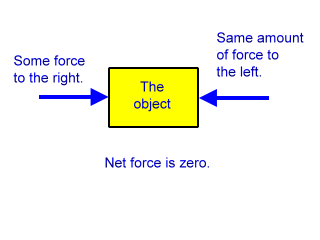Under these conditions the velocity of the object would not change. If it was moving in a straight line at constant speed before the two forces were applied, then it would continue to move in a straight line at constant speed after these two equal and opposite forces were applied. If it was standing still before the application of these forces, it would continue to stand still afterwards.

The net force is the total force. It could be the sum of two forces or more than two forces. If only one force acts upon an object, then this one force would be the net force. If the net force on an object is zero, then the object experiences no velocity change. If the net force on an object is not zero, then the object will show a change in velocity.

Lastly, this net force must be external to the object. The net force can not come from the object itself. You could not, for example, put on ice skates, stand on a frozen pond, push on your back by reaching around with your arms, and expect to get going. Although if someone else came up from behind and gave a you a shove, then your velocity would change.

But skaters do get going all by themselves, so, how does that happen? Well, that answer is in Newton's third law of motion. They push on the ice, and it is the the ice pushing back on them that changes their velocity and gets them going.

Newton's first law of motion contains the same information as Galileo's explanation of inertia.

So, in summary: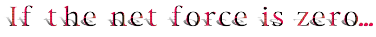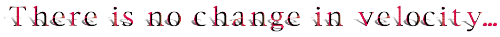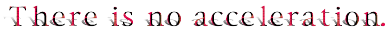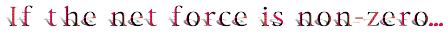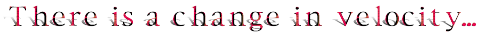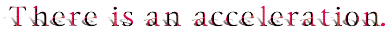Other ways to say Newton's First Law

Click here and link to a collection of other ways to say Newton's First Law. An explanation for each way is included. Hopefully, the explanation will show that each way of saying this law actually describes the same physics.

3-D VRML First Law Demonstration

To see this law in action go to the following VRML 2.0world.

Newton's First Law Demonstration

You will find an object there upon which you can apply a force. You can apply only one force on the object at a time. The object's velocity will only change while this one force is being applied. The VRML world looks like this picture: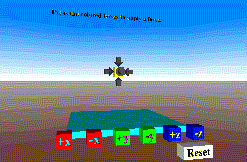The object to be moved floats in the center of the world. There are six dark arrows touching it. Each arrow represents a force that can be activated upon the object. One arrow points in the positive x direction (to the right), another points in the positive y direction (upward), and so on. Click and hold down the mouse on one of the boxes in the foreground to activate the force that is described by the label on the box. The corresponding arrow on the object will turn white, and a force in its direction will be applied.

Notice that with no net force on the object, its velocity does not change. The only way to change the velocity is to apply a non-zero net force. If the object drifts away, either use your browser viewpoint options to get a better look or hit the Reset button. That button will bring the object back to its original position.

Custom Search